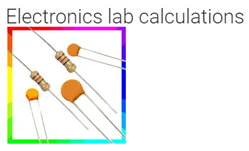# 7 – Best electronic calculator and conversion apps for android and their features

1. Electronics tool kit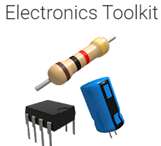This app will be really useful for electronic engineers, students, and hobbyists. This app consists of many calculators such as capacitance calculator, op-amp calculator, DAC and ADC calculator, wavelength frequency calculator, Slew rate calculator, Zener calculator, air core inductor calculator, 555 timer calculator, plate capacitor calculator, resistance to color code calculator, decibel calculator, it can also calculate the wire resistance, and lot more. This app also does the conversion process it has a unit converter for the length, temperature, area, volume, weight, time, angle, power, and base.

2. Electronics engineering calculators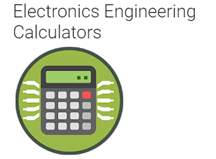This app is very suitable for electronic engineers and students by using this app we can do many calculations and also can design electronic circuits by using this app. In its tool section, we can see many categories like pin-outs, converters, calculators, data sheets IC pins, etc. So this app can be considered as the perfect electronic app. It can do calculations in many categories like inductors, capacitors, voltage regulators, operational amplifiers, AWG calculations, and a lot more.

3. Electronic components and circuit calculator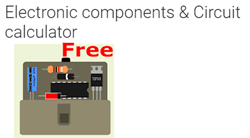This is the perfect app for electrical and electronic students, this is a beginner-friendly app and we can use this to check the electronic component information very easily. This app can also be used to do the circuit calculation, this app has all the electronic components that are needed for a project. The major features of this app are the circuit calculation section, electronics components reference section, and common electronic circuit section. By using the circuit calculator section we can do many calculations like series and parallel resistor calculator, passive butter-worth filter calculator, resistor color code calculator, voltage divider calculator, LCR impedance and resonance calculator, inverting and operational amplifier calculator, delta-star & star-delta calculator, parallel and series RLC calculator and lot more.

4. Electronics circuit calculator-Electronics tool kit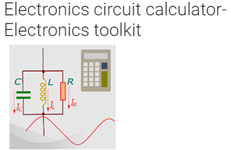This app is powerful and it has many tools, it can do active filter calculations, RF calculations such as a waveguide, attenuators, micro-strip, and frequency. This app will be helpful for circuit prototyping and complex electronic filter design such as butter-worth. By using this app we can do many calculations like transformer turns calculation, current divider calculations, SMD resistor calculator, solenoid calculator, micro-strip calculator, difference amplifier calculator, 555 timer astable multi-vibrator calculations and lot more.

5. Electronics calculator pro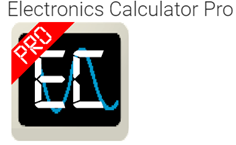By using this app we can do many calculations and conversions, this app also consists of reference tables and pin-outs. With the help of this app, we can do many conversions like angle, temperature, area, power, length, and base. It can also be used to do many calculations like RC and RL circuit calculation, LED resistor calculations, voltage divider calculations, and lot more.

6. Electronics toolboxThis app has basic electronic calculators it can be helpful for electronic engineers and students. It can do many calculations like ohm’s law calculations, RC circuit calculations, RL filter calculations, LC filter calculations, mono-stable and astable circuit calculations, and lot more. It can calculate the resistor values of the voltage divider and can find out combinations of resistance making the desired ratio.